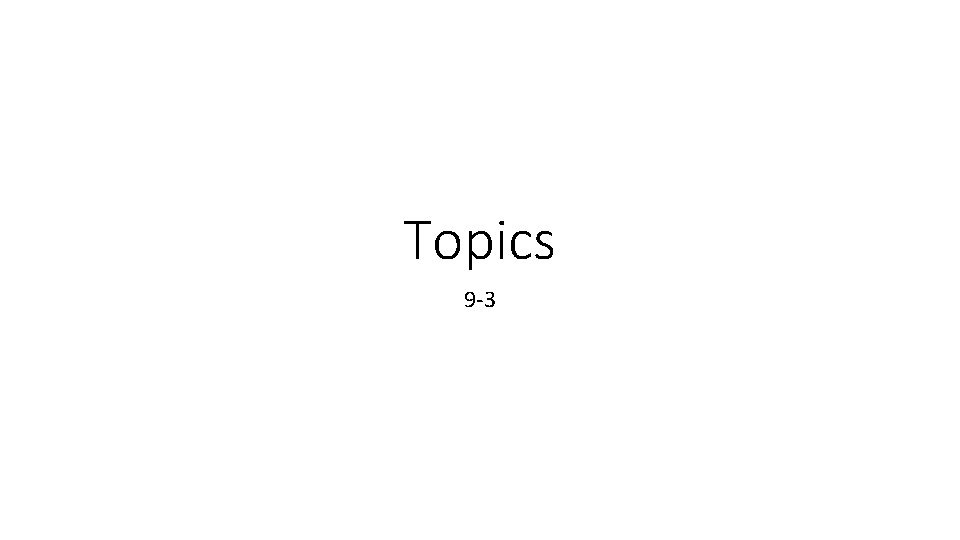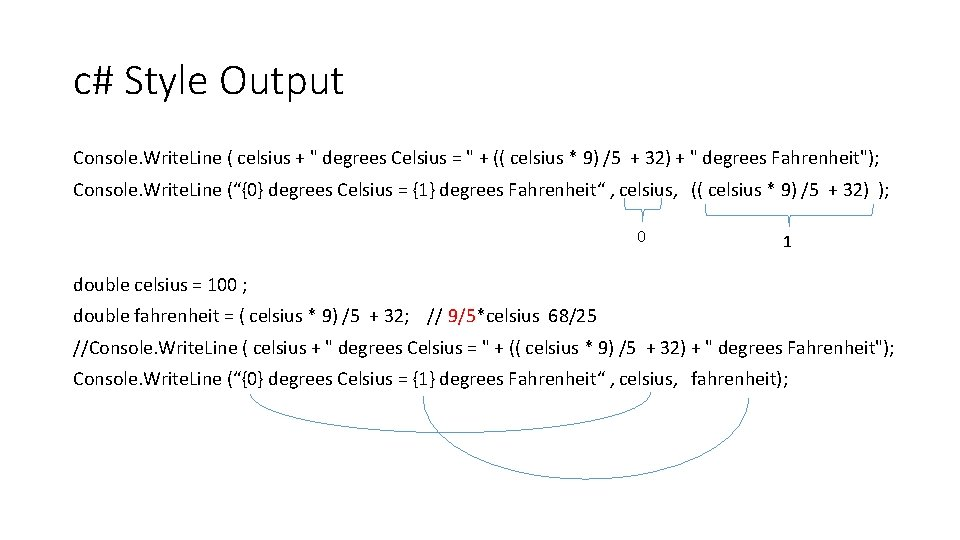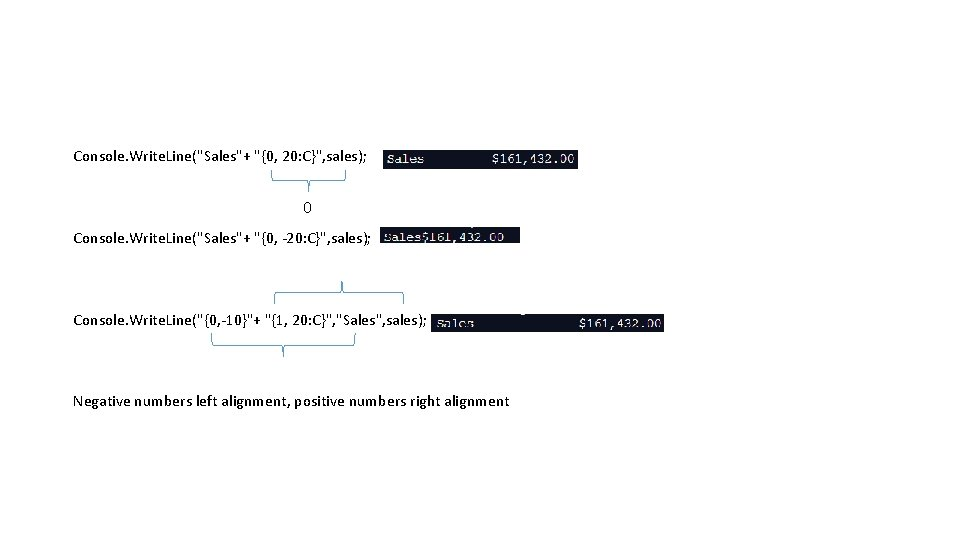# Topics 9 3 c Style Output Console Write

• Slides: 5Topics 9 -3c# Style Output Console. Write. Line ( celsius + " degrees Celsius = " + (( celsius * 9) /5 + 32) + " degrees Fahrenheit"); Console. Write. Line (“{0} degrees Celsius = {1} degrees Fahrenheit“ , celsius, (( celsius * 9) /5 + 32) ); 0 1 double celsius = 100 ; double fahrenheit = ( celsius * 9) /5 + 32; // 9/5*celsius 68/25 //Console. Write. Line ( celsius + " degrees Celsius = " + (( celsius * 9) /5 + 32) + " degrees Fahrenheit"); Console. Write. Line (“{0} degrees Celsius = {1} degrees Fahrenheit“ , celsius, fahrenheit);Console. Write. Line("Sales"+ "{0, 20: C}", sales); 0 Console. Write. Line("Sales"+ "{0, -20: C}", sales); Console. Write. Line("{0, -10}"+ "{1, 20: C}", "Sales", sales); Negative numbers left alignment, positive numbers right alignmentConsole. Write. Line("Cases sold: {0}n. Money Received: {1: C}n. Cost of bars: {2: C}n. Profit: {3: C}n. Donation: {4: C}" , cases, income, bar. Cost, profit, gov. Money); Console. Write. Line("Test Score 2: " + "{0: f 1}", score 2); Format specifier - Location: format Format specifier with Width Specifier – Location, width: formatMagic Numbers • Numbers appearing in your code. • Numbers that are part of a Standard Formulas do not need commented. • loop variables • area of a square • volumes • Magic numbers must be made a constant or commented. • Determining Change will have magic numbers but you should use comments, not constants.